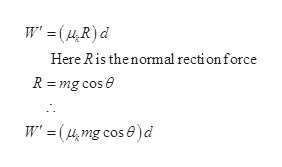# Starting from rest, a 5.00 - kg block slides 2.50 m downa rough 30.0° incline. The coefficient of kinetic frictionbetween the block and the incline is μk = 0.436. Determine(a) the work done by the force of gravity, (b) the work done bythe friction force between block and incline, and (c) the workdone by the normal force. (d) Qualitatively, how would theanswers change if a shorter ramp at a steeper angle were usedto span the same vertical height?

Question
41 views

Starting from rest, a 5.00 - kg block slides 2.50 m down
a rough 30.0° incline. The coefficient of kinetic friction
between the block and the incline is μk = 0.436. Determine
(a) the work done by the force of gravity, (b) the work done by
the friction force between block and incline, and (c) the work
done by the normal force. (d) Qualitatively, how would the
answers change if a shorter ramp at a steeper angle were used
to span the same vertical height?

check_circle

Step 1

a)

the work done by the force of gravity is

Step 2

substitute the given values in the above equation to calculate W

Step 3

b)

the expression for the work done b...help_outlineImage TranscriptioncloseW' =(µ,R)d Here Ris thenormal rection force R = mg cos e W' = (4,mg cos e)d fullscreen

### Want to see the full answer?

See Solution

#### Want to see this answer and more?

Solutions are written by subject experts who are available 24/7. Questions are typically answered within 1 hour.*

See Solution
*Response times may vary by subject and question.
Tagged in

### Physics